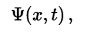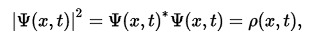# Wave function

A wave function in quantum mechanics is a description of the quantum state of a system. The wave function is a complex-valued probability amplitude, and the probabilities for the possible results of measurements made on the system can be derived from it. in quantum mechanics, variable quantity that mathematically describes the wave characteristics of a particle. The value of the wave function of a particle at a given point of space and time is related to the likelihood of the particle’s being there at the time.

In quantum mechanics, we have a wavefunction, which, as we will see, contains all the information about the system. In general, this wavefunction will be complex. This wavefunction's modulus squared is the probability distribution of the system.

Let us say R is the wavefunction, when the particle is "definitely" Red, and B when it is definitely Blue. So, the general wavefunction, W of the system is a linear combination of the two.

W = xR +yB

where x2 is the probability that the particle is Red. Since this is a two state system, y2 = 1 – x2. The state of such a particle is completely described by its wave function,where x is position and t is time. This is a complex-valued function of two real variables x and t. For one spinless particle in 1d, if the wave function is interpreted as a probability amplitude, the squaremodulus of the wave function, the positive real number.